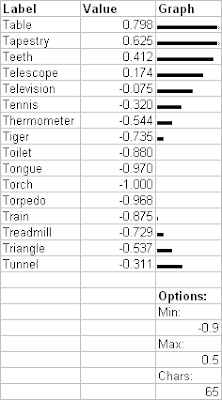## Saturday, April 12, 2008

### An Inline ASCII Bar Chart Technique for Spreadsheets

Here's an article with a neat inline ASCII bar chart technique for spreadsheets.

So, you're a programmer, game designer, artist, producer, or... just about anyone. Chances are you've used Excel. You may even use it to look at data!

Look, here's some generic data (Fig. 1):Wouldn't it be much easier to look at with a chart? Why not build a chart right into the spreadsheet, along side the data (Fig. 2):

Real data may have 100s or 1,000s of rows, with several columns. Being able to skim through the spreadsheet and have the data graphically represented inline is often a big win for readability and locality.

The technique is simple. Excel has a formula to repeat a string several times. For the "Range" graph, the formula is just =REPT("#", D). This works out nicely because the values are integer and small.

The "Value" data takes just a bit more work, but is still trivial to write. Here I've used =REPT("|", F6*20), (Fig. 3)With just a bit more work, we can setup a nice general formula that can be tweaked for each data range, and is more robust. Also, setting the font of the graph to "Small Fonts" with a point size of 4 give us single pixel accuracy in our bar chart, see (Fig. 4).Note the broken bar indicating overflow for the first 2 lines,
the easily configurable min max range,
and the single pixel accuracy of the bars.

Each row of the bar chart uses the formula:
=LEFT(CONCATENATE(REPT("|",E\$57-1),">"),MAX(0,(D34-E\$53)/(E\$55-E\$53)*E\$57))

Let me break it down:

I'm going to substitute names instead of cell references, so it's easier to follow along:
(If you don't know about them, try selecting a cell and using Insert/Name/Define and Insert/Name/Apply some time. But, it's out of the scope of this article)

Ok, the formula is then:
=LEFT(CONCATENATE(REPT("|",Chars-1),">"),MAX(0,(DataValue-Min)/(Max-Min)*Chars))

First, we want to map the data values between Min and Max to appear on the bar chart, so we normalize to 0-1 range:
=LEFT(CONCATENATE(REPT("|",Chars-1),">"),MAX(0,(DataValue-Min)/(Max-Min)*Chars))

Then, multiply by the max number of characters we want a bar chart line to have:
=LEFT(CONCATENATE(REPT("|",Chars-1),">"),MAX(0,(DataValue-Min)/(Max-Min)*Chars))

Then, we want to clamp to positive numbers only, so that data values less than our minimum value don't break our graph:
=LEFT(CONCATENATE(REPT("|",Chars-1),">"),MAX(0,(DataValue-Min)/(Max-Min)*Chars))

That gives us the number of characters to display. But, we would like to visually show when the data values are larger than the bar chart can show. So, instead of taking the number so far and giving it to REPT, we give it to LEFT. Left will take N characters from a string, and we'll terminate that string with a > symbol to show that the graph is maxing out. The equation could be as such:
=LEFT("|||||||||||||||||||||||||||||>",MAX(0,(DataValue-Min)/(Max-Min)*Chars))

But, hard-coding the number of characters on the graph is a draw back. We want to be able to copy and paste this solution around, and scale the graph up as needed. So, we use REPT to generate the |||||||| characters, and concatenate with the final >
=LEFT(CONCATENATE(REPT("|",Chars-1),">"),MAX(0,(DataValue-Min)/(Max-Min)*Chars))

The controls for the graph can be put in the column of the graph above or below the data. We can easily have several columns of bar charts, each with tunable parameters.

I use this technique frequently, I hope you keep it in mind (at least the simple version) the next time you're trolling over data in Excel.